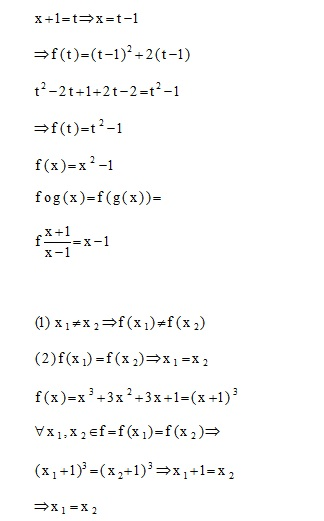# Mathematical Analysis of Theory of Production, Cost and Decisions of the Firms

##### Mathematical Analysis of Theory of Production, Cost and Decisions of the Firms:

If fx+1=x2 = x2 + 2x, so what is fx ? To solve such problems, we use the variance of the variable, that is, we first equate the phrase in parentheses with the variable t and then calculate the x based on and we bring and put in the function:

x+1=t => x = t-1  => f(t)

Regarding this we will have two issues. If the production has increase, the rials and quantitative selling will be of importance. Howsoever the quantitative sales are increased in the domestic and foreign economy, the supply and demands for the Iranian money will be increased and the currency will have an increase not the decrease. In this equation X is a function of production and costs. If the x increases in the domestic and foreign economy, the more Iranian money value will be increased with a relative consistency.0 replies